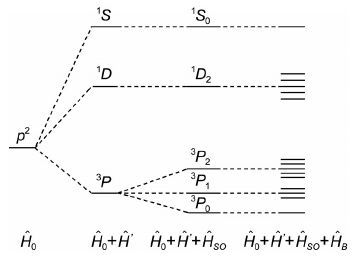## The Structure of Many-Electron Atoms

,,,

 基金资助: 国家基础科学人才培养基金.  J1103306

 Fund supported: 国家基础科学人才培养基金.  J1103306Abstract

In this paper, we represent the structures of many-electron atoms with different approximate Hamiltonian operators. Some related contents such as Slater determinants, electronic configurations, terms and levels are also discussed.

Keywords： Structural chemistry ; Many-electron atoms

CHEN Lan, SUN Hong-Wei, LAI Cheng-Ming. The Structure of Many-Electron Atoms. University Chemistry[J], 2016, 31(7): 91-95 doi:10.3866/PKU.DXHX201509011

## 1 定态Schrödinger方程的解ψ( ${\vec{r}}$ )与原子的状态

$ih\frac{\partial }{\partial t}\psi \left( \vec{r},t \right)=\hat{H}\psi \left( \vec{r},t \right)$

$ih\psi \left( {\vec{r}} \right)\frac{\text{d}f\left( t \right)}{\text{d}t}=f\left( t \right)\hat{H}\psi \left( {\vec{r}} \right)$

$\frac{ih}{f\left( t \right)}\frac{\text{d}f\left( t \right)}{\text{d}t}=\frac{1}{\psi \left( {\vec{r}} \right)}\hat{H}\psi \left( {\vec{r}} \right)$

$\frac{\text{d}f\left( t \right)}{f\left( t \right)}=-\frac{i}{h}E\text{d}t$

$f\left( t \right)=C\exp \left( -\frac{i}{h}Et \right)$

$\hat{H}\psi \left( {\vec{r}} \right)=E\psi \left( {\vec{r}} \right)$

$\psi \left( \vec{r},t \right)=\exp \left( -\frac{i}{h}Et \right)\psi \left( {\vec{r}} \right)$

\begin{align} & \hat{H}\psi \left( \vec{r},t \right)=\hat{H}\left[ f\left( t \right)\psi \left( {\vec{r}} \right) \right]=f\left( t \right)\hat{H}\psi \left( {\vec{r}} \right) \\ & =f\left( t \right)Ef\left( t \right)\psi \left( {\vec{r}} \right)=E\psi \left( \vec{r},t \right) \\ \end{align}

\begin{align} & \psi *\left( \vec{r},t \right)\psi \left( \vec{r},t \right)=\exp \left( \frac{i}{h}Et \right)\psi *\left( {\vec{r}} \right) \\ & \exp \left( -\frac{i}{h}Et \right)\psi \left( {\vec{r}} \right)=\psi *\left( {\vec{r}} \right)\psi \left( {\vec{r}} \right) \\ \end{align}

### 2 采用不同精度的Hamilton算符下多电子原子的结构

\begin{align} & \hat{H}=\sum\limits_{i=1}^{n}{\left( -\frac{{{h}^{2}}}{2m}\nabla _{i}^{2}-\frac{{{e}^{2}}}{4\pi {{\varepsilon }_{0}}{{r}_{ij}}} \right)}+{{{\hat{H}}}_{so}} \\ & =\sum\limits_{i=1}^{n}{\left( -\frac{{{h}^{2}}}{2m}\nabla _{i}^{2}+{{V}_{i}}\left( {{r}_{i}} \right) \right)}+\sum\limits_{i=1}^{n}{\left( -{{V}_{i}}\left( {{r}_{i}} \right)-\frac{Z{{e}^{2}}}{4\pi {{\varepsilon }_{0}}{{r}_{ij}}}+\sum\limits_{j>i}^{n}{\frac{{{e}^{2}}}{4\pi {{\varepsilon }_{0}}{{r}_{ij}}}} \right)} \\ & +{{{\hat{H}}}_{so}}={{{\hat{H}}}_{o}}+{\hat{H}}'+{{{\hat{H}}}_{so}} \\ \end{align}

### 2.1 组态：Ĥ = Ĥ0精度下的多电子原子

$\left( -\frac{{{h}^{2}}}{2m}\nabla _{i}^{2}+{{V}_{i}}\left( {{r}_{i}} \right) \right){{\psi }_{10}}={{\varepsilon }_{i0}}{{\psi }_{10}}$

${{\psi }_{o}}=\frac{1}{\sqrt{n!}}\left| \begin{matrix} {{\psi }_{10}}\left( 1 \right){{\eta }_{1}}\left( 1 \right) & \cdots & {{\psi }_{10}}\left( n \right){{\eta }_{1}}\left( n \right) \\ \vdots & {} & \vdots \\ {{\psi }_{n0}}\left( 1 \right){{\eta }_{1}}\left( 1 \right) & \cdots & {{\psi }_{n0}}\left( n \right){{\eta }_{1}}\left( n \right) \\\end{matrix} \right|$

${{E}_{O}}=\sum\limits_{i=1}^{n}{{{\varepsilon }_{i0}}}\left( {{n}_{i}},{{l}_{i}} \right)$

### 图1## 参考文献 原文顺序 文献年度倒序 文中引用次数倒序 被引期刊影响因子

Levine,I.N.Quantum Chemistry.6th ed.北京:世界图书出版公司,2011.

/

 〈〉